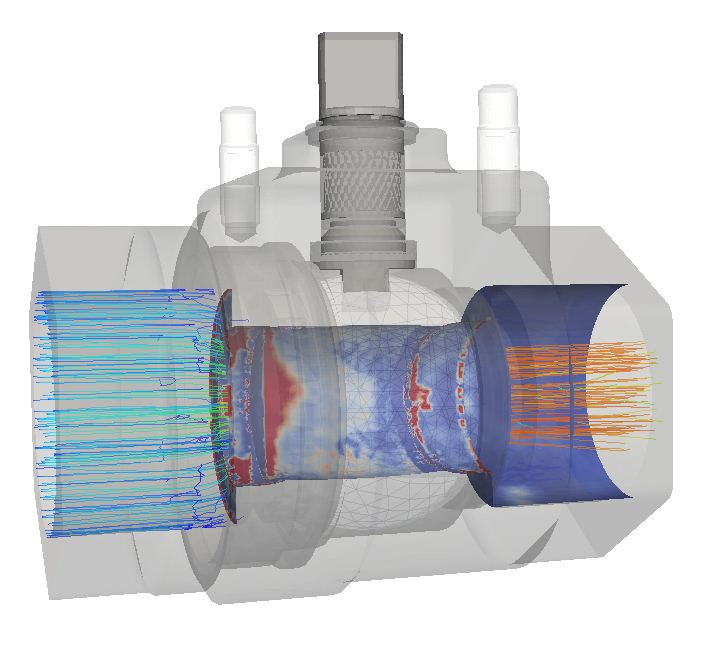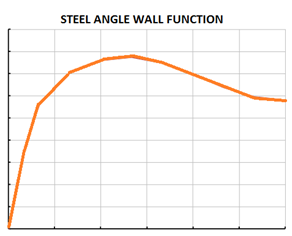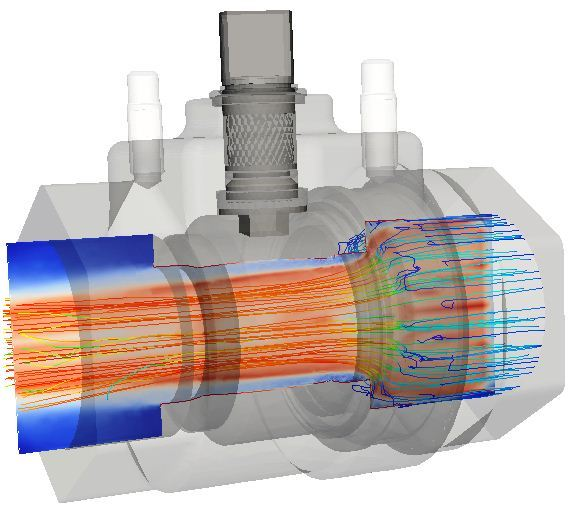# CFD erosion modelling white paperComputational Fluid Dynamics (CFD) is aimed to predict the fluid performance using a computer-based simulation approach. Industrial problems including fluids normally involve complex physics as heat transfer, turbulence and other energy transport mechanisms. The objective of the CFD engineers is to find a representative set of equations that enables the prediction of turbulence behavior of the fluid and take conclusions to adopt successful design decisions.

One demanding problem involving complex physics is erosion. Erosion is the phenomenon of part’s material loss due to mechanical forces induced by solid particles, liquid droplets or even bubbles from cavitation events. Different approaches have been taken historically involving different degrees of complexity. Simpler methods are preferable when available but when the answers are not obvious, and this happens often in erosion prediction, the simplest theories do not always deliver the degree of accuracy required.

Erosion engineer’s objective is to find the most relevant variables that govern the erosion process. The historical evolution of erosion theories starts with basic assumptions involving only fluid variables, and not particle variables like size, density or impact ratios. American institute of petroleum (API) engineers used these fluid variables to obtain a simple expression of the maximum velocity for the fluid inside pipes or other equipment. This expression relates the maximum velocity of the fluid to the density of the fluid and a tuning constant. As a first approach it is acceptable in most cases where simplicity is mandatory, but requirements sometimes oblige to push design a step further and more complex calculations are needed.

The next natural step is to include more variables in the models and create local predictions rather than an overall maximum velocity constrain. Relevant studies in the matter found that the particle impact rate, the impact velocity and the angle are also important variables that have to be considered. This is the base for most of the erosion models currently available in the industry. An erosion rate of the particles can be estimated from these erosion law models. These models make use of the impact velocity and angle of impact estimated from CFD simulations as well as the physical properties of the particles and the valve/pipe. Different parameters for the erosion models can be obtained from international standards (DNV RP O501 – REVISION 4.2 – 2007) for different cases. The expression relates the erosion rate (Kg of material removed by Kg of sand hitting it) and can be represented in his general form like this:

ER=A[W]pnF(α)The most important factors in this expression are the particle velocity variable W and the impact shape function which depends on the material (see figure on the right for an example of a typical steel angle function). N and A parameters are model constants that can be adjusted to match specific cases but guide values are available in literature.

The next question is obvious:

• How can we find the velocity of the particles and the angle function for my specific geometry and flow conditions?

Well, this is where numerical simulations come to play a very important role. CFD tools and the experienced PRE Technologies' team can provide reliable models to predict these functions and get an accurate view on the expected erosion rates for complex geometries under different environments.

Currently most CFD erosion studies use a three-stage approach:

• The flow is modeled first
• Particle trajectories are calculated explicitly (DPM model)
• Erosion damage is obtained using erosion laws
Variations of these stages can also be performed, like coupling particles to the fluid in system with high loads of sand for example.The fluid flow calculation is common for most of the CFD models, but the second stage involving particles is by far the least conventional in terms of computational fluid dynamics. An Euler-Lagrange approach is assumed using the Navier Stokes equations for the fluid and kinematic equilibrium equations for the particles.

The fluid state is used to calculate the fluid-particle interaction and to obtain the balance of forces which ultimately enables the calculation of the trajectory of the particles assuming the Newton equilibrium principles.

The identification and quantification of the forces acting on the particle are far from being simple and many different forces can influence the particle path. As an example, we could mention drag and lift forces depending on geometric properties, buoyant terms and even turbulence fluid fluctuations can disperse them and change their expected trajectory. All these terms are included in a CFD model and an accurate track of the particles can be obtained.

Once the particles position time histories are obtained, interaction with walls must be calculated to obtain the impact velocity and angles, also restitution coefficients must be set to include non-elastic effects of the collisions. Once this task is over, the prediction of erosion rate is completed and the results provide a rate of degradation of the material in terms of depth of material loss per unit of time, typically mm per year.

PRE Technologies’ background in erosion includes the exercise of comparison between different models and finding the most suitable for each application. Do not hesitate to contact us and our team will shed some light on your erosion problems.

Keep your curiosity in good shape.